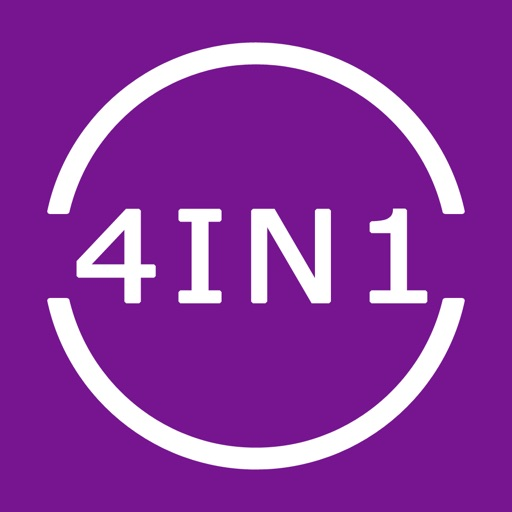## Everything you need to convert, calculate, simplify and compare fractions in one app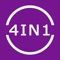# Fraction Converter Calculator

by Intemodino Group s.r.o.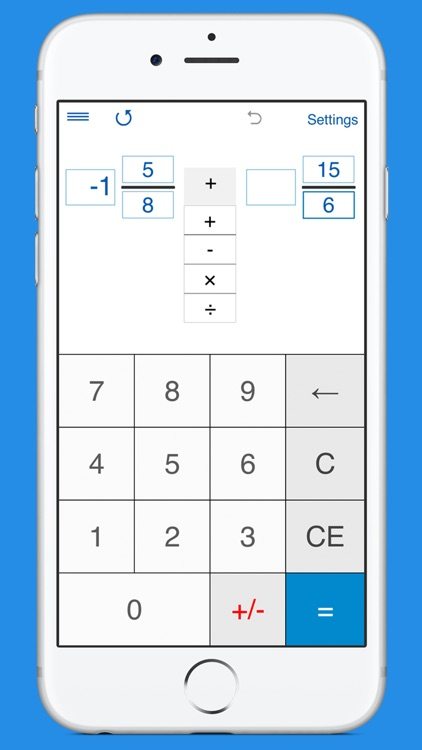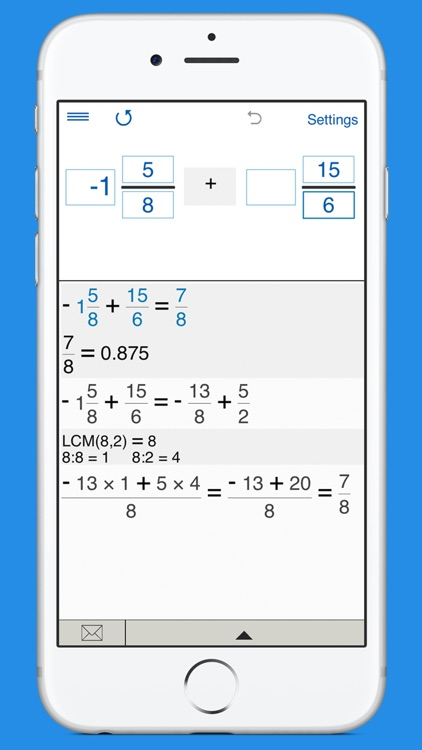Everything you need to convert, calculate, simplify and compare fractions in one app!### App Details

Version
4.2.1
Rating
(22)
Size
25Mb
Genre
Education Utilities
Last updated
October 8, 2018
Release date
April 26, 2013

### App Screenshots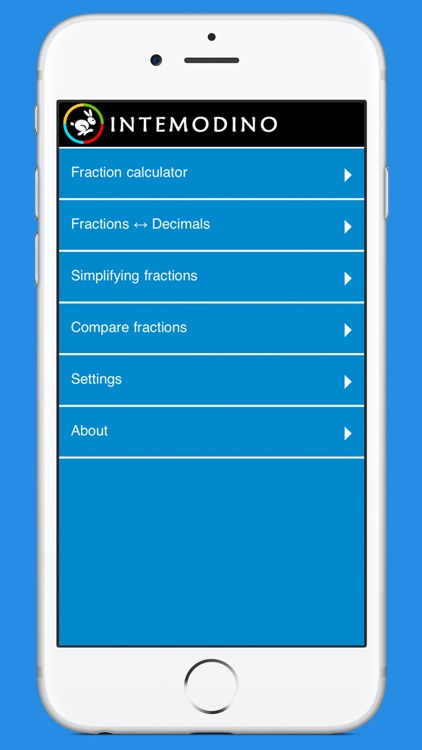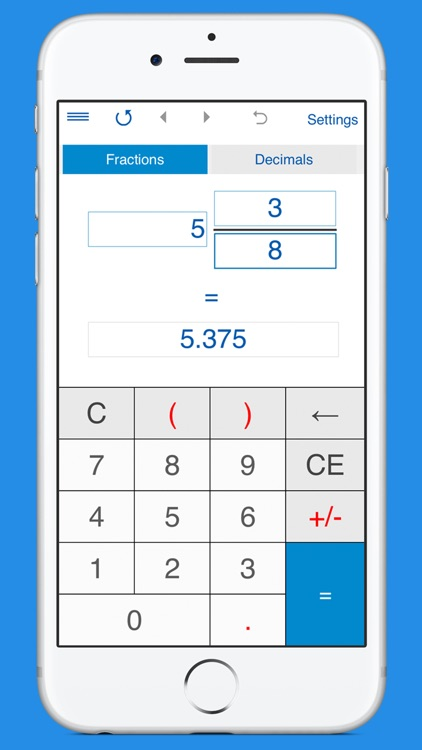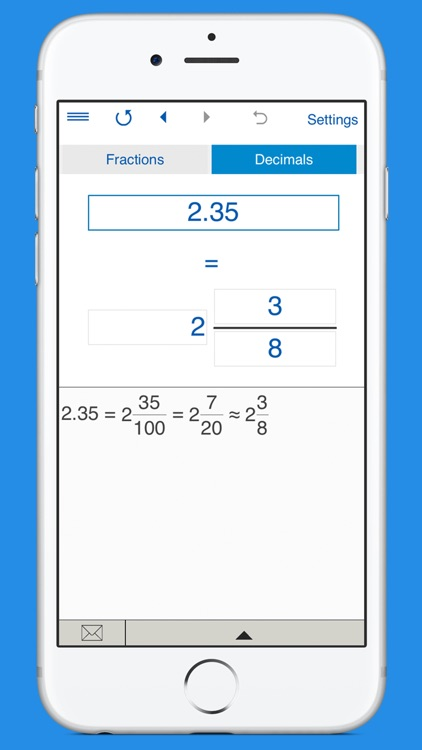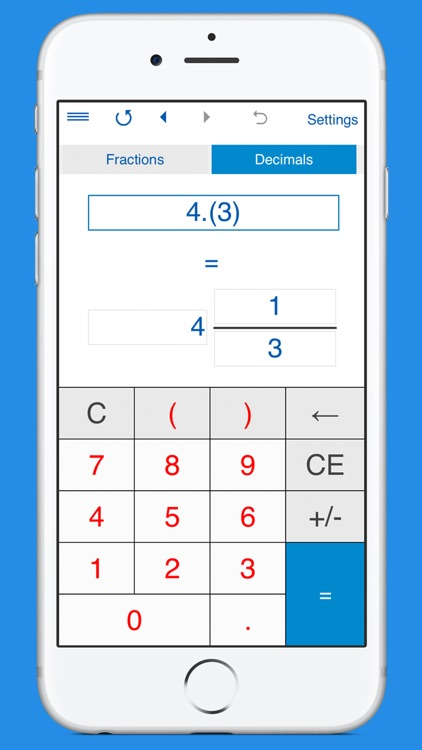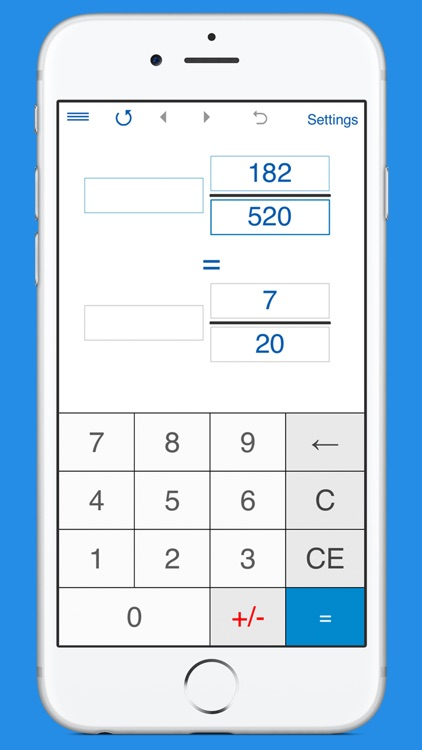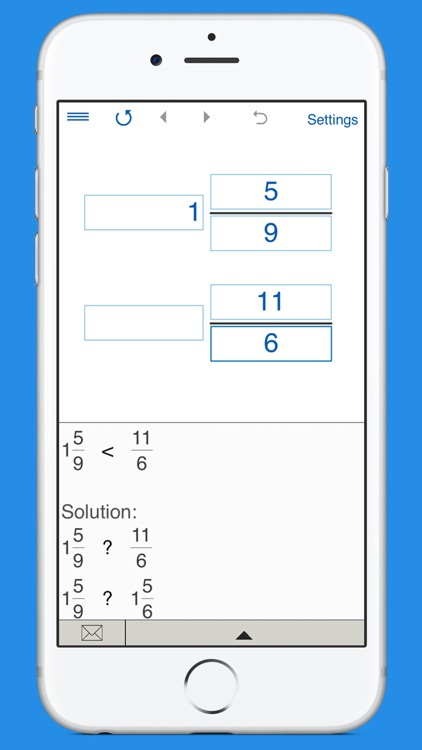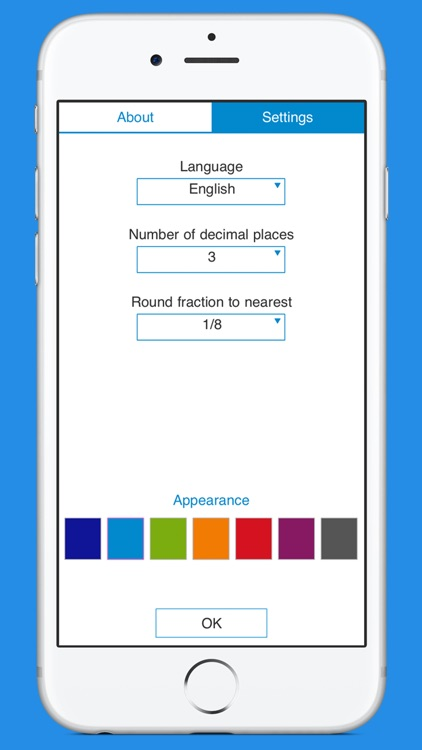### App Store Description

Everything you need to convert, calculate, simplify and compare fractions in one app!

Fraction Converter and Calculator is perfect for engineers, carpenters, builders, students and anyone who needs a comprehensive fraction app.

► FRACTION CONVERTER
- Converts fractions to decimals and vice versa.
- Supports proper and improper fractions, mixed numbers, terminating and repeating decimals.
- To convert a fraction to a decimal number, tap ‘Fractions', input a fraction or a mixed number and tap "=".
- To convert a decimal number to a fraction, tap ‘Decimals’, enter a decimal number using the decimal point "." as separator (eg. 1.23) and tap "=".
You can use this converter for both terminating decimals and repeating decimals.
For repeating decimals enclose the repeating digits in parenthesis. Eg. type 0.24(3) for 0.24333... If the number is 5.123123..., type 5.(123).
- Round fractions to the nearest 1/2, 1/4, 1/8, 1/16, 1/32, 1/64, 1/128, 1/256.

► FRACTION CALCULATOR
- Fraction calculator with steps for two and three fractions.
- Performs basic operations (addition, subtraction, multiplication, and division) with proper and improper fractions, mixed numbers and whole numbers. Supports negative values.
- To add, subtract, multiply, and divide three fractions, you should select a landscape orientation.
- Round fractions to the nearest 1/2, 1/4, 1/8, 1/16, 1/32, 1/64, 1/128, 1/256.

► FRACTION SIMPLIFIER
Calculator for reducing fractions and mixed numbers.

► COMPARE FRACTIONS CALCULATOR
The app compares proper and improper fractions and mixed numbers.
You can compare two or three fractions. To compare three fractions, you should select a landscape orientation.

OTHER FEATURES:
• Shows step-by-step solution.
• History tape to view your recent calculations.
• Back and forward buttons to check or recall recent calculations.
• Sends results and history via email.
• 'Undo' for the Clear command.
• Personalize the look and feel of the app by changing the color of the theme.
• The app supports both portrait and landscape orientation.

APP'S SETTINGS:
- Rounding results to the desired number of decimal places. To round results to the nearest whole number, select 0 decimal places.
- In Fraction Calculator and Fraction Converter, you can select to round fractions to the nearest 1/2, 1/4, 1/8, 1/16, 1/32, 1/64, 1/128, 1/256. By default, the app rounds fractions to the nearest 1/32.
If you do not need to round fractions, just select "-".
- 7 color schemes.
- Supports 18 languages:
Chinese, Czech, Danish, Dutch, English, Finnish, French, German, Greek, Italian, Japanese, Korean, Norwegian, Portuguese, Russian, Spanish, Swedish, Turkish.

EVERYTHING YOU NEED FOR TEACHING AND LEARNING FRACTIONS
Fraction calculator and converter by Intemodino Group helps you solve various fraction problems.
Learn and practice how to add, subtract, multiply, divide, compare, and simplify fractions. In addition, you can learn how to convert fractions to decimals and decimals to fractions.
Just choose the fraction problem you want to solve from the list.

TOPICS COVERED:
2. Multiplying Fractions.
3. Dividing Fractions.
4. Comparing and Ordering Fractions.
5. Simplifying Fractions.
6. Converting Fractions to Decimals.
7. Converting Decimals to Fractions.